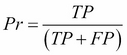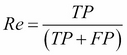•#### Learning Bayesian Models with R#### Overview of this book

Learning Bayesian Models with RCreditswww.PacktPub.comPrefaceFree Chapter
Introducing the Probability TheoryThe R EnvironmentIntroducing Bayesian InferenceMachine Learning Using Bayesian InferenceBayesian Regression ModelsBayesian Classification ModelsBayesian Models for Unsupervised LearningBayesian Neural NetworksBayesian Modeling at Big Data ScaleIndex## Performance metrics for classification

To understand the concepts easily, let's take the case of binary classification, where the task is to classify an input feature vector into one of the two states: -1 or 1. Assume that 1 is the positive class and -1 is the negative class. The predicted output contains only -1 or 1, but there can be two types of errors. Some of the -1 in the test set could be predicted as 1. This is called a false positive or type I error. Similarly, some of the 1 in the test set could be predicted as -1. This is called a false negative or type II error. These two types of errors can be represented in the case of binary classification as a confusion matrix as shown below.

Confusion Matrix

Predicted Class

Positive

Negative

Actual Class

Positive

TP

FN

Negative

FP

TN

From the confusion matrix, we can derive the following performance metrics:

• Precision:This gives the percentage of correct answers in the output predicted as positive
• Recall:This gives the percentage...